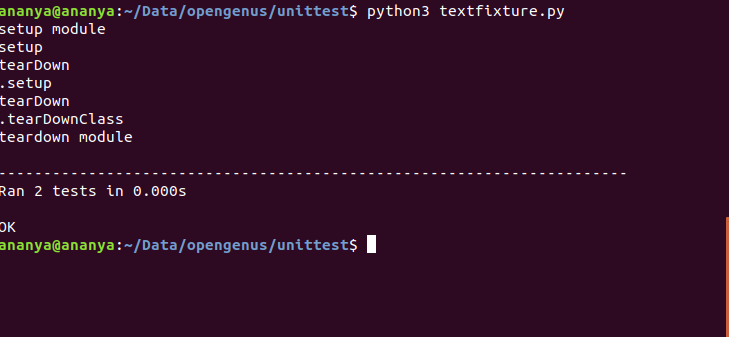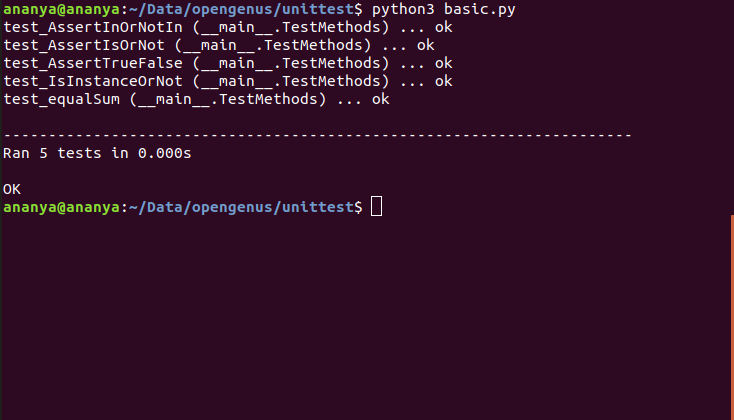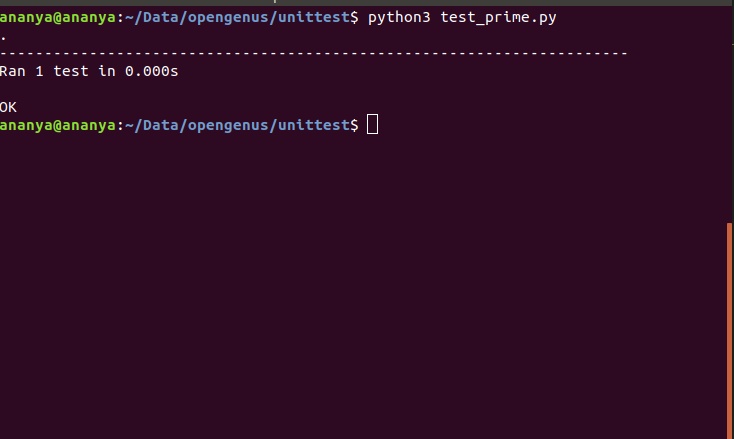×

Search anything:

# Introduction to Unit Testing in Python with unittest module

#### Software Engineering PythonIndian Technical Authorship Contest starts on 1st July 2023. Stay tuned.

Reading time: 30 minutes | Coding time: 10 minutes

In this article we will understand how to use unit testing in Python and implement a demo using unittest module and master its use.

What is Software Testing?

Software Testing is the process of evaluating the functionality of software applications with an intent to find whether the application is meeting the specific requirements or not.
It's done to ensure that the application is defect free.

Software Testing involves following levels of testing: (lower to higher precedence)

1. Unit Testing (done by developers)
2. Integration Testing (done by testers)
3. System Testing (done by testers)
4. Application Testing (done by end user)

What is Unit Testing?

Unit Testing is the beginner level in Software Testing where individual units or components of the applications are tested. In Object Oriented Programming, the smallest unit is method. It is used to validate individual units of the software performs as designed.

What is unittest module in Python?
Just like any other unit testing framework like NUnit framework (for C#), JUNit (for Java), unittest is the testing framework for Python language.

Following are the unittest components to support OOPs concept:

• test fixture - is used as a baseline needed to perform tests. This involves creating proxy databases, starting a server.
• test case - set of conditions and checks specific response for it.
• test suite - collection of testcases used to test and show specific behaviours by executing aggregated tests together.
• test runner - used to setup the tests and provides the outcome to the user.

Finding it difficult to understand the above words? Let's understand them with the help of examples.

• Test Fixture - In easy words, Test fixtures are the resources i.e. methods and functions that run before and after a test. Basically, those methods are used to setup the appropriate resources and testing environment.

Common fixture methods are setUp() and tearDown()

• setUp() - runs prior the testing process ensuring proper setup of the environment.
• tearDown() - runs after the completion of testing process.

The above methods can be omitted if there's no need for specific initializations (setUp()) or cleaups (tearDown()).

``````import unittest
import inspect

def fibonacci(n):
return 1 if n<=2 else fibonacci(n-1)+fibonacci(n-2)

def setUpModule():
print("setup module")

def tearDownModule():
print("teardown module")

class TestFibonacci(unittest.TestCase):

def setUp(self):

#setting up the value for n
print("setup")
self.n = 10

def tearDown(self):

#clean up task by deleting n
print("tearDown")
del self.n

@classmethod
def setupClass(cls):
print("setup class")

@classmethod
def tearDownClass(cls):
print("tearDownClass")

def test_fibonacci(self):
self.assertEqual(fibonacci(self.n),55)

def test_fibonacci_true(self):
self.assertTrue(fibonacci(self.n)==55)

if __name__ == '__main__':
unittest.main()
``````From the above code, Let's understand the flow of the testing.

• The setup for the value "n" was done at the setUp().
• Then the 2 test methods namely test_fibonacci() and test_fibonacci_true() excecuted.
• At last, tearDown() function was called for the clean up task.
• "Ran 2 tests in 0.000s" - 2 tests here refers to the tests test_fibonacci() and test_fibonacci_true().

Fun Tasks for the beginners and anyone :p

• try deleting both the setUp() and tearDown() methods and try to observe the output.
• try deleting only the setUp() method and observe the output.

Did you notice any difference? I hope the above two tasks will get you the clarity for the setUp() and tearDown() methods.

Lets understand the basics of unittest framework with code

``````import unittest

class Animal:

def print():
cout<<"It's an animal"

class Fruit:

def print():
cout<<"Apple is a fruit"

cat = Animal()
apple = Fruit()

class TestMethods(unittest.TestCase):

def test_equalSum(self):
self.assertEqual(sum([2,3,4]),9,"Should be 9")
self.assertNotEqual(sum([2,3,4]),7,"Not Equal Assert Function")

def test_AssertTrueFalse(self):
self.assertTrue('OPENGENUS'.isupper())
self.assertFalse('Opengenus'.islower())

def test_AssertIsOrNot(self):
self.assertIs(4,4)
self.assertIsNot(4,5)

def test_AssertInOrNotIn(self):
self.assertIn(2,[2,3,4])
self.assertNotIn(2,[3,4,5])

def test_IsInstanceOrNot(self):

#passing cat object of Animal class
self.assertIsInstance(cat,Animal)

#passing apple object of Fruit class and checking with Animal class
self.assertNotIsInstance(apple, Animal)

if __name__ == '__main__':
unittest.main(verbosity=2)

``````• assertEqual(first,second,msg=None)/assertNotEqual(first,second,msg=None) - used to check if the result obtained is equal to the expected result.
• assertTrue(expr, msg=None)/assertFalse(expr, msg=None) - used to verify if a given statement is true or false.
• assertRaise() - used to raise a specific exception.
• assertIsInstance(first,second,msg=None)/assertNotIsInstance(first,second,msg=None) - used to check that obj is or is not an instance of the class respectively.
• assertIn(first,second,msg=None)/assertNotIn(first,second,msg=None) - used to test whether the first parameter is present in the second parameter or not.

Let's go through the code and the respective result.

In the first line of the output you may notice ".F"
Let's understand the meaning of it.

The possible outcomes of unit testing are as follows:

1. '.' = equivalent to "All Test Passed".
2. 'F' = equivalent to "Test didnt pass successfully"
3. 'E' = equivalent to "Error"

Overrall result was Failed because of the following line:

``````self.assertEqual(sum([2,3,4]),6,"False")
``````

Let's write a seperate test file for an existing code.

Include following lines to the file primenumbers.py

``````def is_prime(number):
"""Returns True if number is prime"""

if number<0:
return False

if number == 1 or number == 0:
return False
for element in range(number):
if number % element == 0:
return False

return True
``````

Include the following lines to the file testprime.py

``````import unittest

class PrimeorNot(unittest.TestCase):

def test_prime(self):
self.assertTrue(is_prime(7))
self.assertFalse(is_prime(9))
self.assertFalse(is_prime(-11))
self.assertFalse(is_prime(1))

if __name__ == '__main__':
unittest.main()

``````Unit Testing is simple no? Indeed !!

Important Things to keep in mind

• Don't ignore unit testing just by seeing the word testing. It's the thing which tells you about how the application works. (a linkage between frontend and backend). And it's done by Developers to test the working of the unit modules.

• If you want to make your software product work efficiently and excellent, Testing is a must.

For getting deeper insights into unit testing in python: Refer Python Documentation.
https://docs.python.org/2/library/unittest.html#